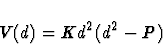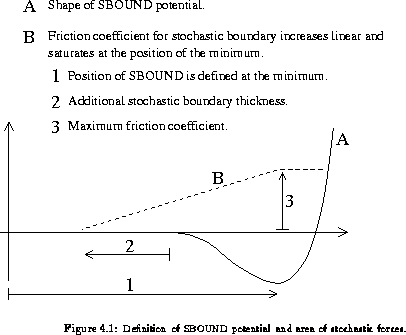Next: Maximum Friction Coefficient Up: The Control File Previous: Switch for SHAKE on

# Definition of SBOUND Region

SBOUND forces  restrain molecules to a given volume. The forces at the edge of the volume are designed to mimic the effects of solvent water. In EGO cubical and spherical boundaries can be defined (even simultaneously). The faces of a cube are defined by the positions of planes which are parallel to the coordinate system. Spheric SBOUNDs are defined by center and radius. A value of zero for a face position or for the radius indicates that no corresponding SBOUND will be used.

Curvature of SBOUND edges'' sets the radius in Å joining two or three SBOUND planes. If set to zero, SBOUND planes are joined rectangularly and you can select single planes, e.g., only infinite parallel XZ planes. If you have a positive radius, all planes will be active, even if they contain the origin. For details of SBOUND definition see also Figure 4.1.

Usually SBOUND is used together with stochastic boundary. Thus stochastic boundary should be set to TRUE and a Maximum friction coefficient'' greater then zero should be specified.

If any SBOUND is used, individual friction factors defined in coord.lis are disregarded. However, individual harmonic restraints can still be specified in addition to SBOUND if the switch for harmonic restraints is also set to TRUE.

EGO uses the SBOUND-potentialwith the constants K=0.2kcal/mol/Å4 and P=2.25Å. These constants are defined in the constants file ego.h.Next: Maximum Friction Coefficient Up: The Control File Previous: Switch for SHAKE on
Helmut Heller
2000-04-19